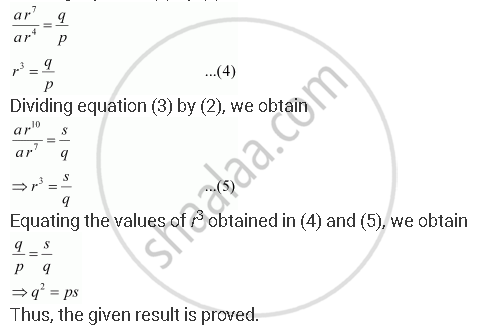Share

# The 5th, 8th and 11th Terms of a G.P. Are P, Q and S, Respectively. Show that Q2 = Ps. - Mathematics

#### Question

The 5th, 8th and 11th terms of a G.P. are pq and s, respectively. Show that q2 = ps.

#### Solution

Let a be the first term and r be the common ratio of the G.P.

According to the given condition,

a5 = a r5–1 a r4 = p … (1)

aa r8–1 a r7 = q … (2)

a11 = a r11–1 a r10 = s … (3)

Dividing equation (2) by (1), we obtainIs there an error in this question or solution?